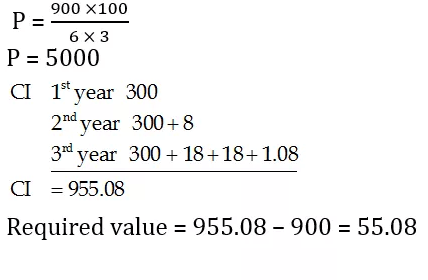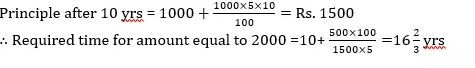# Quant Quiz for RRB PO & SBI CLERK MAINS 2019 | 22 July 2019

## Quant Quiz for RRB PO & SBI CLERK MAINS

Quant Quiz to improve your Quantitative Aptitude for SBI Po & SBI clerk exam IBPS PO Reasoning , IBPS Clerk Reasoning , IBPS RRB Reasoning, LIC AAO ,  and other competitive exams.

Q1. A bag contains 5 red balls and 7 blue balls. Two balls are drawn at random without replacement, and then find the probability of that one is red and other is blue.

A) 33/65

B) 35/66

C)  37/66

D) 41/65

E) None of these

Answer & Explanation
Q1. Ans(B)Q2. A bag contains 3 red balls and 8 blacks ball and another bag contains 5 red balls and 7 blacks balls, one ball is drawn at random from either of the bag, find the probability that the ball is red.

A) 93/264

B) 95/264

C) 91/264

D) 97/264

E) None of these

Answer & Explanation
Q2. Ans(C)Q3.  12 persons are seated at a circular table. Find the probability that 3 particular persons always seated together.

A) 9/55

B) 7/55

C) 4/55

D) 3/55

E) None of these

Answer & Explanation
Q3. Ans(D)

Taking 3 particular persons as single entity, we have 12 – 3 + 1 = 10 persons to be seated.Q4.  P and Q are two friends standing in a circular arrangement with 10 more people. Find the probability that exactly 3 persons are seated between P and Q.

A) 5/11

B) 4/11

C) 2/11

D)  3/11

E) None of these

Answer & Explanation
Q4. Ans(C)Q5.  A basket contains 5 black and 8 yellow balls. Four balls are drawn at random and not replaced. What is the probability that they are of different colours alternatively.

A) 56/429

B) 57/429

C) 61/429

D) 68/429

E) None of these

Answer & Explanation
Q5. Ans(A)Q6. A man borrows Rs. 4000 at 20% compound rate of interest. At the end of each year he pays back Rs. 1500. How much amount should he pay at the end of the third year to clear all his dues ?

(a) Rs. 2592

(b) Rs. 2852

(c) Rs. 2952

(d) Rs. 2953

(e) None of these

Answer & Explanation
Q6. Ans(c)[/su_spoiler]

Q7. Ashok borrowed some money at simple interest at the rate of 6 per cent per annul for the first two years, at the rate of 9 per cent per annul for the next three years and at the rate of 14% per cent per annul for the period beyond five years. If he pays a total interest of Rs. 11400 at the end of 9 years how much money did he borrow ?

(a) Rs. 16,000

(b) Rs. 14,000

(c) Rs. 18,000

(d) Rs. 12,000

(e) None of these

Answer & Explanation
Q7. Ans(d)Q8. On Rs 3500 invested at a simple interest rate 7 per cent per annum, Rs 500 is obtained as interest in certain years, In order to earn Rs 800 as interest on Rs 4900 in the same number of years, what should be the rate of simple interest?

(a) 9%

(b) 10%

(c) 12%

(d) 8%

(e) None of these

Answer & Explanation
Q8. Ans(d)Q9. A person invested a certain amount at simple interest at the rate of 6 per cent per annul earning Rs. 900 as an interest at the end of three years. Had the interest been compounded every year, how much more interest would he have earned on the same amount with the same interest rate after three years ?

(a) Rs. 38.13

(b) Rs. 25.33

(c) Rs. 55.08

(d) Rs. 35.30

(e) None of these

Answer & Explanation
Q9. Ans(c)Q10. If Rs.1000 be invested at interest rate of 5% and the interest be added to the principle every 10 years, then In how many years  it will amount to Rs. 2000 ?Answer & Explanation
Q10. Ans(a)### You Can Read This Also:

Best E-books for LIC AAO 2019 : Get PDF here

ambitiousbaba.com need your support to Grow

I challenge you will get Best Content in Our PDFs with Detail solutions and Latest Pattern

Memory Based Puzzle E-book | 2016-19 Exams Covered

Get PDF here

Caselet Data Interpretation 200 Questions

Get PDF here
Puzzle & Seating Arrangement E-Book for BANK PO MAINS (Vol-1)

Get PDF here

### ARITHMETIC DATA INTERPRETATION 2019 E-book

Get PDF here
The Banking Awareness 500 MCQs E-book| Bilingual (Hindi + English)

Get PDF here

High Level DATA INTERPRETATION Practice E-BOOK

Get PDF here

### How to Access on App:-

1. Go to Playstore search Ambitious Baba or Click here to Install App
2. After Install Login with Google Account or Facebook Account